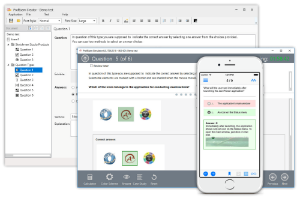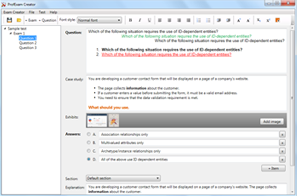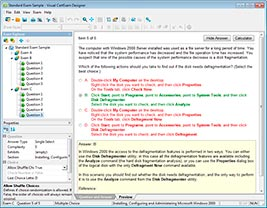# Download Introduction to Programming Using Python.PracticeTest.98-381.2018-08-10.1e.20q.vcex

## File Info

 Exam Introduction to Programming Using Python Number 98-381 File Name Introduction to Programming Using Python.PracticeTest.98-381.2018-08-10.1e.20q.vcex Size 769 Kb Posted August 10, 2018 Downloads 101

### How to open VCEX & EXAM Files?

Files with VCEX & EXAM extensions can be opened by ProfExam Simulator.

Coupon: EXAMFILESCOM
With discount: 20%

## Demo Questions

Question 1
The ABC company has hired you as an intern on the coding team that creates e-commerce applications.
You must write a script that asks the user for a value. The value must be used as a whole number in a calculation, even if the user enters a decimal value.
You need to write the code to meet the requirements.
Which code segment should you use?

• A: totalItems = input(“How many items would you like?”)
• B: totalItems = float(input(“How many items would you like?”))
• C: totalItems = str(input(“How many items would you like?”))
• D: totalItems = int(input(“How many items would you like?”))

Question 2
During school holidays, you volunteer to explain some basic programming concepts to younger siblings.
You want to introduce the concept of data types in Python. You create the following three code segments:You need to evaluate the code segments.
For each of the following statements, select Yes if the statement is true. Otherwise, select No.
NOTE: Each correct selection is worth one point.

Question 3
The ABC company is converting an existing application to Python. You are creating documentation that will be used by several interns who are working on the team.
You need to ensure that arithmetic expressions are coded correctly.
What is the correct order of operations for the six classes of operations ordered from first to last in order of precedence? To answer, move all operations from the list of operations to the answer area and arrange them in the correct order.

Question 4
You are writing a Python program. The program collects customer data and stores it in a database.
The program handles a wide variety of data.
You need to ensure that the program handles the data correctly so that it can be stored in the database correctly.
Match the data type to the code segment. To answer, drag the appropriate data type from the column on the left to its code segment on the right. Each data type may be used once, more than once, or not at all.

Question 5
You are creating a Python program that shows a congratulation message to employees on their service anniversary.
You need to calculate the number of years of service and print a congratulatory message.
You have written the following code. Line numbers are included for reference only.You need to complete the program.
Which code should you use at line 03?

• A: print(“Congratulations on” + (int(end)-int(start)) + “years of service!”)
• B: print(“Congratulations on” + str(int(end)-int(start)) + “years of service!”)
• C: print(“Congratulations on” + int(end - start) + “years of service!”)
• D: print(“Congratulations on” + str(end - start)) + “years of service!”)

Question 6
You are writing a Python program to perform arithmetic operations.
You create the following code:What is the result of each arithmetic expression? To answer, drag the appropriate expression from the column on the left to its result on the right. Each expression may be used once, more than once, or not at all.

Question 7
You are writing a Python program that evaluates an arithmetic formula.
The formula is described as b equals a multiplied by negative one, then raised to the second power, where a is the value that will be input and b is the result.
You create the following code segment. Line numbers are included for reference only.You need to ensure that the result is correct.
How should you complete the code on line 02? To answer, drag the appropriate code segment to the correct location. Each code segment may be used once, more than once, or not at all. You may need to drag the split bar between panes or scroll to view content.
NOTE: Each correct selection is worth one point.

Question 8
Evaluate the following Python arithmetic expression:What is the result?

• A: 3
• B: 13
• C: 15
• D: 69

Question 9
You develop a Python application for your company.
A list named employees contains 200 employee names, the last five being company management. You need to slice the list to display all employees excluding management.
Which two code segments should you use? Each correct answer presents a complete solution. (Choose two.)

• A: employees [1:-4]
• B: employees [:-5]
• C: employees [1:-5]
• D: employees [0:-4]
• E: employees [0:-5]

Question 10
You are an intern for ABC electric cars company. You must create a function that calculates the average velocity of their vehicles on a 1320 foot (1/4 mile) track. The output must be as precise as possible.
How should you complete the code? To answer, select the appropriate code segments in the answer area.

CONNECT US##### PROFEXAM WITH A 20% DISCOUNT

You can buy ProfExam with a 20% discount..

HOW TO OPEN VCEX AND EXAM FILES

Use ProfExam Simulator to open VCEX and EXAM filesHOW TO OPEN VCE FILES

Use VCE Exam Simulator to open VCE files